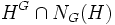# Pronormal not implies NE

This article gives the statement and possibly, proof, of a non-implication relation between two subgroup properties. That is, it states that every subgroup satisfying the first subgroup property (i.e., pronormal subgroup) need not satisfy the second subgroup property (i.e., NE-subgroup)
View a complete list of subgroup property non-implications | View a complete list of subgroup property implications
EXPLORE EXAMPLES YOURSELF: View examples of subgroups satisfying property pronormal subgroup but not NE-subgroup|View examples of subgroups satisfying property pronormal subgroup and NE-subgroup

## Statement

A pronormal subgroup of a group need not be a NE-subgroup.

## Proof

### Examples of Sylow subgroups

The proof that pronormal subgroups need not be NE follows from facts (1) and (2). Further, any example of a Sylow subgroup that is not NE gives an example of a pronormal subgroup that is not NE. Two examples of situations where Sylow subgroups are not NE are the$2$-Sylow subgroup and the$5$-Sylow subgroup in the alternating group of degree five.

Further information: Sylow not implies NE

### Example of the symmetric group

Further information: symmetric group:S4

Let$G$ be the symmetric group on the set$\{ 1,2,3,4 \}$, and$H$ be the four-element subgroup$\{ (), (1,2,3,4), (1,3)(2,4), (1,4,3,2) \}$. Then,$H$ is a pronormal subgroup, because the subgroup generated by$H$ and any other conjugate of it is the whole group. On the other hand, we have$H^G = G$ and$N_G(H)$ is a dihedral group of order eight, so$H^G \cap N_G(H)$ is a dihedral group of order eight, which is strictly bigger than$H$.